Tamilnadu State Board New Syllabus Samacheer Kalvi 12th Maths Guide Pdf Chapter 11 Probability Distributions Ex 11.6 Textbook Questions and Answers, Notes.

## Tamilnadu Samacheer Kalvi 12th Maths Solutions Chapter 11 Probability Distributions Ex 11.6

Choose the most suitable answer from the given four alternatives:

Question 1.
Let X be random variable with probability density functionWhich of the following statement is correct?
(a) Both mean and variance exist
(b) Mean exists but variance does not exist
(c) Both mean and variance do not exist
(d) Variance exists but Mean does not exist
Solution:
(b) Mean exists but variance does not exist
Hint:Question 2.
A rod of length 2l is broken into two pieces at random. The probability density function of the shorter of the two pieces isThe mean and variance of the shorter of the two pieces are respectively
(a) $$\frac { l }{ 2 }$$, $$\frac { l^2 }{ 3 }$$
(b) $$\frac { l }{ 2 }$$, $$\frac { l^2 }{ 6 }$$
(c) l, $$\frac { l^2 }{ 12 }$$
(d) $$\frac { l }{ 2 }$$, $$\frac { l^2 }{ 12 }$$
Solution:
(d) $$\frac { l }{ 2 }$$, $$\frac { l^2 }{ 12 }$$
Hint: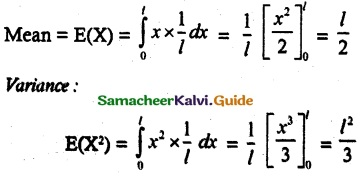V(X)= E(X²) – [E(x)]²
$$\frac { l^2 }{ 3 }$$ – $$\frac { l^2 }{ 4 }$$ = $$\frac { l^2 }{ 12 }$$Question 3.
Consider a game where the player tosses a six-sided fair die. If the face that comes up is 6, the player wins Rs 36, otherwise he loses Rs k², where k is the face that comes up k = {1, 2, 3, 4, 5}. The expected amount to win at this game in Rs is
(a) $$\frac { 19 }{ 6 }$$
(b) –$$\frac { 19 }{ 6 }$$
(c) $$\frac { 3 }{ 2 }$$
(d) –$$\frac { 3 }{ 2 }$$
Solution:
(b) –$$\frac { 19 }{ 6 }$$
Hint:
Probability mass functionQuestion 4.
A pair of dice numbered 1, 2, 3, 4, 5, 6 of a six-sided die and 1, 2, 3, 4 of a four-sided die is rolled and the sum is determined. Let the random variable X denote this sum. Then the number of elements in the inverse image of 7 is
(a) 1
(b) 2
(c) 3
(d) 4
Solution:
(d) 4
Hint:
X-1 (7) = {(3,4), (4, 3), (5, 2), (6, 1)} = 4Question 5.
A random variable X has binomial distribution with n = 25 and p = 0.8 then standard deviation of X is
(a) 6
(b) 4
(c) 3
(d) 2
Solution:
(d) 2
Hint:
n = 25, p = $$\frac { 4 }{ 5}$$, q = $$\frac { 1 }{ 5 }$$
SD = $$\sqrt { npq }$$
= $$\sqrt { 25 × \frac { 4 }{ 5} × \frac { 1 }{ 5} }$$
= 2

Question 6.
Let X represent the difference between the number of heads and the number of tails obtained when a coin is tossed n times. Then the possible values of X are
(a) i + 2n, i = 0, 1, 2 …, n
(b) 2i – n, i = 0, 1, 2 …, n
(c) n – i, i = 0, 1, 2 …, n
(d) 2i + 2n, i = 0, 1, 2 …, n
Solution:
(b) 2i – n, i = 0, 1, 2 …, n
Hint:
if n = 1 then x = 0,1
if n = 2 then x = -2, 0, 2
if n = 3 then x = -3, -1, 1, 3
Let x = 2i – n
when n = 2
x = 2i – 2; i = 0, 1, 2
i = 0 ⇒ x = -2
i = 1 ⇒ x = 0
i = 2 ⇒ x = 2
x = -2, 0, 2
when n =3
x = 2i – 3; i = 0, 1, 2, 3
i = 0 ⇒ x = 3
i = 1 ⇒ x = -1
i = 2 ⇒ x = 1
i = 3 ⇒ x = 3
x = -3, -1, 1, 3
∴ 2i – n, i = 0, 1, 2, ……. n.Question 7.
If the function f(x) = $$\frac { 1 }{ 12 }$$ for a < x < b represents a probability density function of a continuous random variable X, then which of the following cannot be the value of a and b?
(a) 0 and 12
(b) 5 and 17
(c) 7 and 19
(d) 16 and 24
Solution:
(d) 16 and 24
Hint:Question 8.
Four buses carrying 160 students from the same school arrive at a football stadium. The buses carry, respectively, 42, 36, 34, and 48 students. One of the students is randomly selected. Let X denote the number of students that were on the bus -carrying the randomly selected student. One of, the 4 bus drivers is also randomly selected. Let Y denote the number of students on that bus. Then E[X] and E[Y] respectively are
(a) 0.11
(b) 1.1
(c) 11
(d) 1
Solution:
(b) 1.1
Hint: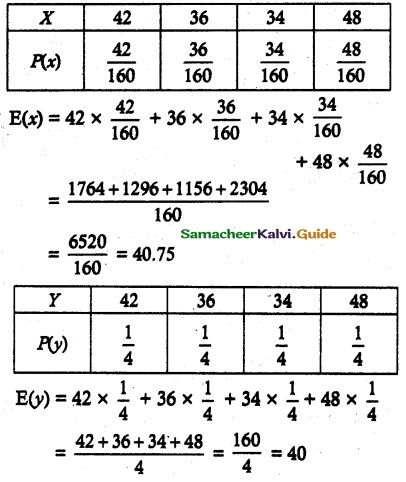Question 9.
Two coins are to be flipped. The first coin will land on heads with probability 0.6, the second with Probability 0.5. Assume that the results of the flips are independent and let X equal the total number of heads that result. The value of E[X] is
(a) 50, 40
(b) 40, 50
(c) 40.75, 40
(d) 41, 41
Solution:
(c) 40.75, 40
Hint:
P(H1) = 0.6 P(H1) = 0.4
P(H2) = 0.5 P(H2) = 0.5
Random variable x = 0, 1, 2
P(x = 0) = P(H1) P(H2)
= 0.4 × 0.5 = 0.2
P(x = 1) = P(H1).P(H2) + P(H2).P(H1)
= 0.6 × 0.5 + 0.5 × 0.4
= 0.3 + 0.2 = 0.5
P(X = 2) = P(H1).P(H2)
= 0.6 × 0.5 = 0.3E(x) = 0 × 0.2 + 1 × 0.5 + 2 × 0.3
= 0 + 0.5 + o.6
= 1.1

Question 10.
On a multiple-choice exam with 3 possible destructives for each of the 5 questions, the probability that a student will get 4 or more correct answers just by guessing is
(a) $$\frac { 11 }{ 243 }$$
(b) $$\frac { 3 }{ 8 }$$
(c) $$\frac { 1 }{ 243 }$$
(d) $$\frac { 5 }{ 243 }$$
Solution:
(c) $$\frac { 11 }{ 243 }$$
Hint:Question 11.
If P(X = 0) = 1 – P(X = 1). If E[X] = 3 Var(X), then P(X = 0) is
(a) $$\frac { 2 }{ 3 }$$
(b) $$\frac { 2 }{ 5 }$$
(c) $$\frac { 1 }{ 5 }$$
(d) $$\frac { 1 }{ 3 }$$
Solution:
(d) $$\frac { 1 }{ 3 }$$
Hint:
P(X = 0) = 1 – P(x = 1)
Let P(x = 0) = a
1 – P(X = 1) = a
P(X = 1) = 1 – a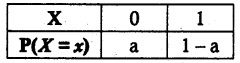E(x) = 0 × a + 1 × 1 – a
= 0 + 1 – a
= 1 – a
E(x²) = 0² × a + 1² × 1 – a
= 1 – a
Var x = E(x²) – (E(x))²
= 1 – a – (1 – a)²
= 1 – a – (1 – 2a + a²)
= 1 – a – 1 + 2a – a²
= a – a²
E(x) = 3 var (x)
1 – a = 3 (a – a²)
1 – a = 3a – 3a²
3a² – 4a + 1 = 0
(3a – 1)(a – 1) = 0
a = $$\frac { 1 }{ 3 }$$ a = 1
P(X = 0) = a = $$\frac { 1 }{ 3 }$$

Question 12.
If X is a binomial random variable with I expected value 6 and variance 2.4, Then P(X = 5) isSolution:
(d)
Hint:
E(X) = np = 6, Var(x) = npq = 2.4Question 13.
The random variable X has the probability density function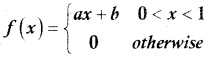and E(X) = $$\frac { 7 }{ 12 }$$ then a and b are respectively
(a) 1 and $$\frac { 1 }{ 2 }$$
(b) $$\frac { 1 }{ 2 }$$ and 1
(c) 2 and 1
(d) 1 and 2
Solution:
(a) 1 and $$\frac { 1 }{ 2 }$$
Hint:Question 14.
Suppose that X takes on one of the values 0, 1 and 2. If for some constant k, P(X = i) = kP(X = i – 1) for i = 1, 2 and P(X = 0) = $$\frac { 1 }{ 7 }$$. Then the value of k is
(a) 1
(b) 2
(c) 3
(d) 4
Solution:
(b) 2
Hint:
P(X = 0) = $$\frac { 1 }{ 7 }$$
P(X = i) = k P(X = i – 1)
i = 1
P(X = 1) = k P(X = 0)
= $$\frac { k }{ 7 }$$
i = 2
P(X = 2) = k P(X = 1)
= k($$\frac { k }{ 7 }$$) = $$\frac { k^2 }{ 7 }$$
Probability mass functionΣ p(x) = 1
$$\frac { 1 }{ 7 }$$ + $$\frac { k }{ 7 }$$ + $$\frac { k^2 }{ 7 }$$ = 1
1 + k + k² = 7
k² + k – 6 = 0
(k + 3)(k – 2) = 0
k = -3 (Not possible); k = 2
∴ k = 2Question 15.
Which of the following is a discrete random variable?
I. The number of cars crossing a particular signal in a day.
II. The number of customers in a queue to buy train tickets at a moment.
III. The time taken to complete a telephone call.
(a) I and II
(b) II only
(c) III only
(d) II and III
Solution:
(a) I and II

Question 16.
Ifis a probability density function of a random variable, then the value of a is
(a) 1
(b) 2
(c) 3
(d) 4
Solution:
(a) 1
Hint:
f is p.d.f $$\int_{0}^{a}$$
$$\int_{0}^{a}$$ 2x dx = 1
[x²]$$_{0}^{a}$$ = 1
a² = 1
a = 1Question 17.
The probability mass function of a random variable is defined as: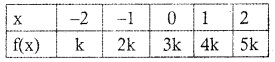Then E(X) is equal to:
(a) $$\frac { 1 }{ 15 }$$
(b) $$\frac { 1 }{ 10 }$$
(c) $$\frac { 1 }{ 3 }$$
(d) $$\frac { 2 }{ 3 }$$
Solution:
(d) $$\frac { 2 }{ 3 }$$
Hint:
f(x) is p.m.f, Σ f(x) = 1
k + 2k + 3k + 4k + 5k = 1
15k = 1
k = $$\frac { 1 }{ 15 }$$
E(X) = -2k -2k + 0 + 4k + 10k
= 10 k
= 10 × $$\frac { 1 }{ 15 }$$ = $$\frac { 2 }{ 3 }$$

Question 18.
Let X have a Bernoulli distribution with a mean of 0.4, then the variance of (2X – 3) is
(a) 0.24
(b) 0.48
(c) 0.6
(d) 0.96
Solution:
(d) 0.96
Hint:
p = 0.4
q = 1 – p
= 0.6
Var (x) = pq
= 0.4 × 0.6
= 0.24
Var (2x – 3) = 2² var(x)
= 4 var(x)
= 4(0.24)
= 0.96Question 19.
If in 6 trials, X is a binomial variable which follows the relation 9P(X = 4) = P(X = 2), then the probability of success is
(a) 0.125
(b) 0.25
(c) 0.375
(d) 0.75
Solution:
(b) 0.25
Hint:
9 P(X = 4) = P(X = 2)
9 x 6C4 p4 q² = 6C2 p² q4
9p² = q²
9p² = 1- 2p + p²
8P² + 2p – 1 = 0
p = $$\frac { 1 }{ 4 }$$ = 0.25

Question 20.
A computer salesperson knows from his past experience that he sells computers to one in every twenty customers who enter the showroom. What is the probability that he will sell a computer to exactly two of the next three customers?
(a) $$\frac { 57 }{ 20^3 }$$
(b) $$\frac { 57 }{ 20^2 }$$
(c) $$\frac { 19^3 }{ 20^3 }$$
(d) $$\frac { 57 }{ 20 }$$
Solution:
(a) $$\frac { 57 }{ 20^3 }$$
Hint: